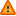FAQ/euclid - CBU statistics WikiYou should trust both wikis because the password could be read by the particular administrators.

Clear message
location: FAQ / euclid

# What is Euclidean distance and how do I compute it ?

Euclidean distance measures the distance between two vectors of length t denoting t traits of various observations and is a specific example of Mahalanobis distance with an identity covariance matrix (ie uncorrelated traits).

ED = for vectors, observations with vectors $$x_text{i} = (x_text{1i}, ..., x_text{ti})text{T}$$ and $$x_text{j} = (x_text{1j}, ..., x_text{tj})text{T}$$ equals $$\sqrt{(x_text{i} - x_text{j})^text{T}(x_text{i} - x_text{j})}$$

This can be written in long hand as $$\sqrt{(x_text{1i}-x_text{1j})text{2} + .. + (x_text{ti}-x_text{tj})text{2}}$$

The Euclidean distance is the distance on a graph between two points. This is easily seen in two dimensions since by Pythagoras's theorem the linear distance (hypotenuse) between two points (x11, x21) and (x12, x22) equals the square root of the squared difference in x and y co-ordinates = square root of (x11-x12)(x11-x12) + (x21-x22)(x22-x21). See here.

The Euclidean distance is a special case of Mahalanobis distance which is used for measuring multivariate group distances or distance of an observation from its group means ie with 2 or more predictors. In particular it is the square root of the Mahalanobis distance, termed D2 in Tabachnick and Fidell (2007), with the covariance matrix replaced by the identity matrix. D2 is defined in, for example, Campbell, Donner and Webster (1991). The Mahalanobis distance can be thought of as a multivariate extension of the square of the z value (where z is the difference in a pair of group means divided by the pooled group standard deviation). It follows that the Euclidean distance is equal to D using the identity matrix.

References

Campbell MK, Donner, A and Webster, KM (1991) Are ordinal models useful for classification? Statistics in Medicine 10 383-394.

Tabachnick BG and Fidell LS (2007) Using multivariate statistics. Pearson International:Boston.